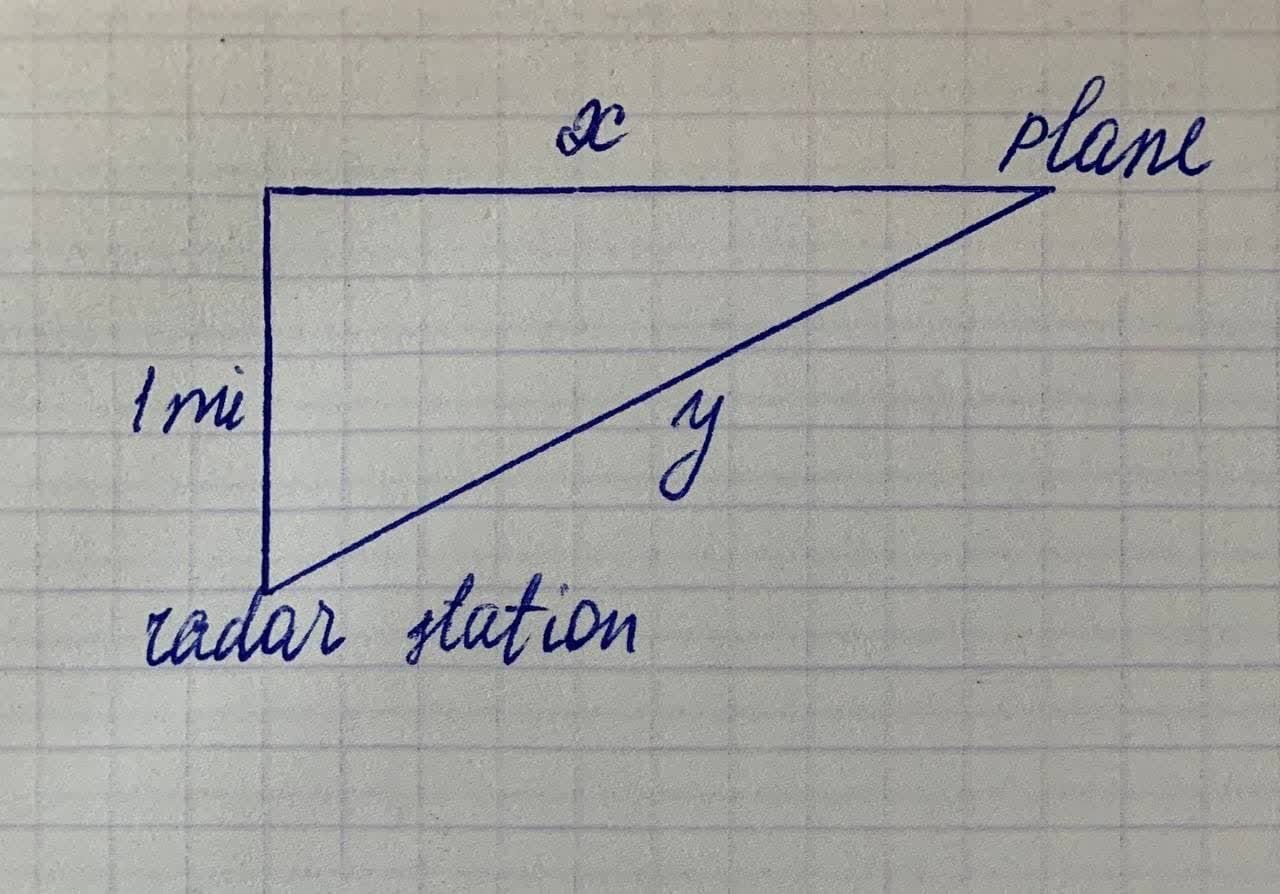Mabel Breault

2021-12-30

A plane flies directly over a radar station while traveling at $500\frac{mi}{h}$ passes directly over a radar station. Find the rate at which the distance from the plane to the station is increasing when it is 2 mi away from the station.
Finish solving the problemJenny Bolton

Note that y is the distance from the plane to the station the problem is referring to.Differentiate with respect to time t the equation that relates everything.
${x}^{2}+{1}^{2}={y}^{2}$
$2x\frac{dx}{dt}+0=2y\frac{dy}{dt}$
$x\frac{dx}{dt}=y\frac{dy}{dt}$
$\frac{x}{y}\frac{dx}{dt}=\frac{dy}{dt}$
We are given that a $\frac{dx}{dt}=500\frac{mi}{h}$. We also need to find x at the moment $y=2mi$
${x}^{2}+{1}^{2}={2}^{2}$
${x}^{2}=4-1=3$
$x=\sqrt{3}$ (ignore negative root)
Plug the values into the differentiated equation.
$\frac{dy}{dt}=\frac{x}{y}\frac{dx}{dt}$
$\frac{\sqrt{3}}{2}\cdot 500$
$250\sqrt{3}\frac{mi}{h}$levurdondishav4

P is the planesVasquez

The appropriate diagram here is a right triangle with a short vertical leg of length 1 representing the distance between the station and a point 1 mi directly above it, a longer horizontal leg of variable length x representing the distance from the point above to the position of the plane at time t, and a hypotenuse connecting the plane’s
position with the station (call this distance y). We're told that $\frac{dx}{dt}=500$. By the Pythagorean Theorem $1+{x}^{2}={y}^{2}$. Differentiating with respect to t and using our rules and formulas
$2\frac{dy}{dt}=\frac{d{y}^{2}}{dy}\frac{dy}{dt}=\frac{d{y}^{2}}{dt}=\frac{d\left(1+{x}^{2}\right)}{dt}$
$=\frac{d1}{dt}+\frac{d{x}^{2}}{dt}=0+\frac{d{x}^{2}}{dx}\frac{dx}{dt}=2x\frac{dx}{dt}$
Now setting y=2 in the equation $1+{x}^{2}={y}^{2}$ gives $x=\sqrt{3}$. So at this point,
$4\frac{dy}{dt}=2\sqrt{3}\frac{dx}{dt}=2\sqrt{3}500$
so that $\frac{dy}{dt}=250\sqrt{3}$ (in miles per hour).

Do you have a similar question?